# Science, Nature & Math Mathematics Books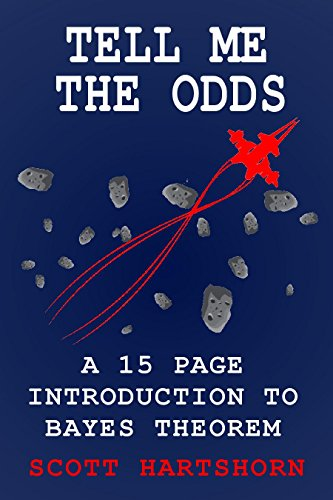## Tell Me The Odds: A 15 Page Introduction To Bayes Theorem

Bayes Theorem Is ImportantBayes Theorem is a way of updating probability as you get new information. Essentially, you make an initial guess, and then get more data to improve it. Bayes Theorem, or Bayes Rule, has a ton of real world applications, from estimating your risk of a heart attack to making recommendations on NetflixBut It Isn't That ComplicatedThis book is a short introduction to Bayes Theorem. It is only 15 pages long, and is intended to show you how Bayes Theorem works as quickly as possible. The examples are intentionally kept simple to focus solely on Bayes Theorem without requiring that the reader know complicated probability distributions.If you want to learn the basics of Bayes Theorem as quickly as possible, with some easy to duplicate examples, this is a good book for you.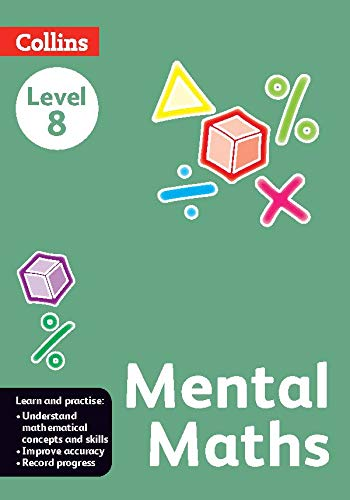## Mental Maths Coursebook 8

Collins Mental Maths is a series of six books available from Level 1 to Level 8 which is linked to the curriculum requirement of each primary group as per NCF guidelines.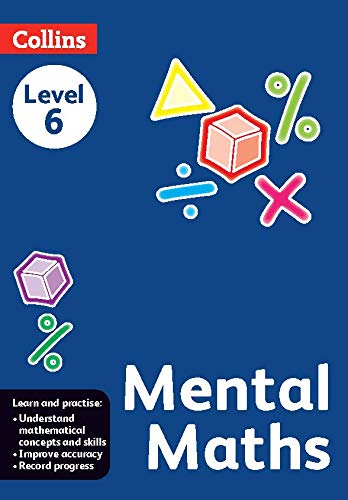## Mental Maths Coursebook 6

Collins Mental Maths is a series of six books available from Level 1 to Level 8 which is linked to the curriculum requirement of each primary group as per NCF guidelines.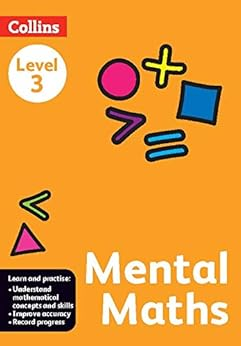## Mental Maths Coursebook 3

Collins Mental Maths is a series of six books available from Level 1 to Level 8 which is linked to the curriculum requirement of each primary group as per NCF guidelines.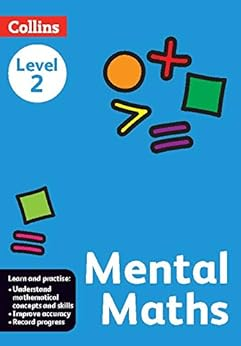## Mental Maths Coursebook 2

Collins Mental Maths is a series of six books available from Level 1 to Level 8 which is linked to the curriculum requirement of each primary group as per NCF guidelines.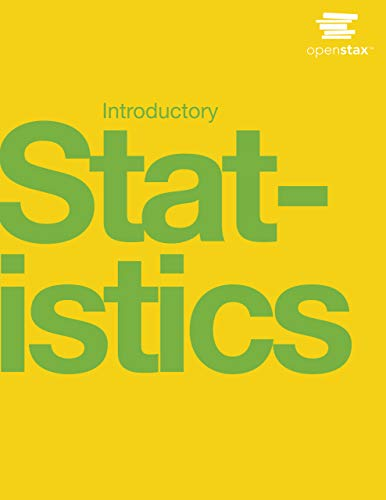## Introductory Statistics

Introductory Statistics follows scope and sequence requirements of a one-semester introduction to statistics course and is geared toward students majoring in fields other than math or engineering. The text assumes some knowledge of intermediate algebra and focuses on statistics application over theory. Introductory Statistics includes innovative practical applications that make the text relevant and accessible, as well as collaborative exercises, technology integration problems, and statistics labs.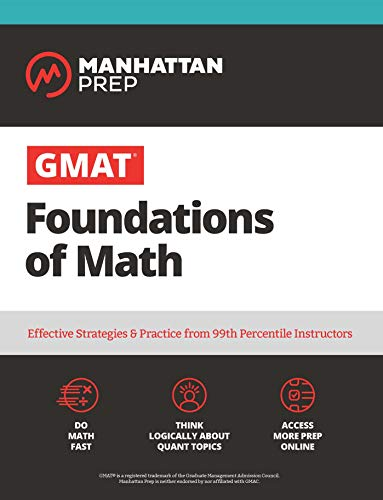## GMAT Foundations of Math: 900+ Practice Problems in Book and Online (Manhattan Prep GMAT Strategy Guides)

Developed for test-takers who need a refresher, Manhattan Prep's GMAT Foundations of Math provides a user-friendly review of basic math concepts crucial for GMAT success.GMAT Foundations of Math comes with robust online resources, including a practice test, question banks, and interactive video lessons. Written by active instructors with 99th-percentile scores, this guide is designed with the student in mind.Designed to be user-friendly for all students, GMAT Foundations of Math provides:700+ practice problems for realistic reviewEasy-to-follow explanations of fundamental math conceptsStep-by-step application of concepts to example problemsGMAT Foundations of Math is an invaluable resource for any student who wants to cement their understanding and build their basic math skills for the GMAT.